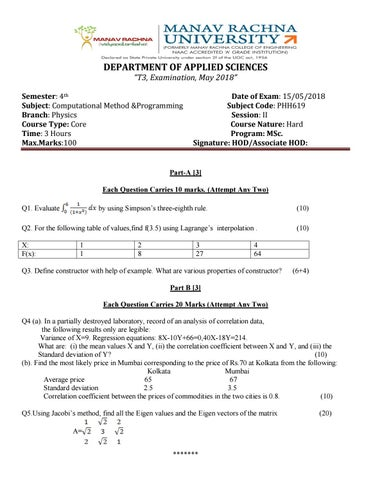DEPARTMENT OF APPLIED SCIENCES “T3, Examination, May 2018” Semester: 4th Subject: Computational Method &Programming Branch: Physics Course Type: Core Time: 3 Hours Max.Marks:100

Date of Exam: 15/05/2018 Subject Code: PHH619 Session: II Course Nature: Hard Program: MSc. Signature: HOD/Associate HOD:

Part-A  Each Question Carries 10 marks. (Attempt Any Two) by using Simpson’s three-eighth rule.

Q1. Evaluate

(10)

Q2. For the following table of values,find f(3.5) using Lagrange’s interpolation . X: F(x):

1 1

2 8

3 27

(10)

4 64

Q3. Define constructor with help of example. What are various properties of constructor?

(6+4)

Part B  Each Question Carries 20 Marks (Attempt Any Two) Q4 (a). In a partially destroyed laboratory, record of an analysis of correlation data, the following results only are legible: Variance of X=9. Regression equations: 8X-10Y+66=0,40X-18Y=214. What are: (i) the mean values X and Y, (ii) the correlation coefficient between X and Y, and (iii) the Standard deviation of Y? (10) (b). Find the most likely price in Mumbai corresponding to the price of Rs.70 at Kolkata from the following: Kolkata Mumbai Average price 65 67 Standard deviation 2.5 3.5 Correlation coefficient between the prices of commodities in the two cities is 0.8. (10) Q5.Using Jacobi’s method, find all the Eigen values and the Eigen vectors of the matrix A= *******

(20)

Q7.Apply Runge-Kutta fourth order method to find an approximate value of y at y(0.2), y(0.4) and y(0.6) Given that dy/dx=1+y2, and y=0 when x=0. (20)

Part C- Each Question Carries 20 Marks (Attempt Any Two) Q6. (i) What is Exception? Explain the Exception handling mechanism of C++ with suitable example. ( 3+5+6) (ii) What is friend function in C++. Write the properties of friend function? (2+4) Q8. (i) What is the difference between derived class and base class? (5) (ii) What is the concept of overriding in C++? (5) (iii) Define Inheritance .What are the different types of Inheritance? What is the advantage of using Inheritance? (2+6+2) Q9. (i) How would you resolve ambiguity in multiple Inheritance and single Inheritance? (10) (ii) What are abstract classes? (2) (iii) What is virtual function? why do we use virtual function? Explain with the help of example. (2+2+4)

*******